# Existence of multiple positive solutions for fourth-order boundary value problems in Banach spaces

## Abstract

This paper deals with the positive solutions of a fourth-order boundary value problem in Banach spaces. By using the fixed-point theorem of strict-set-contractions, some sufficient conditions for the existence of at least one or two positive solutions to a fourth-order boundary value problem in Banach spaces are obtained. An example illustrating the main results is given.

MSC:34B15.

## 1 Introduction

In this paper, we consider the existence of multiple positive solutions for the fourth-order ordinary differential equation boundary value problem in a Banach space E

$\left\{\begin{array}{c}{x}^{\left(4\right)}\left(t\right)=f\left(t,x\left(t\right),-{x}^{″}\left(t\right)\right),\phantom{\rule{1em}{0ex}}t\in \left(0,1\right),\hfill \\ x\left(0\right)=x\left(1\right)={x}^{″}\left(0\right)={x}^{″}\left(1\right)=\theta ,\hfill \end{array}$
(1.1)

where $f:I×E×E\to E$ is continuous, $I=\left[0,1\right]$, θ is the zero element of E. This problem models deformations of an elastic beam in equilibrium state, whose two ends are simply supported. Owing to its importance in physics, the existence of this problem in a scalar space has been studied by many authors using Schauder’s fixed-point theorem and the Leray-Schauder degree theory (see  and references therein). On the other hand, the theory of ordinary differential equations (ODE) in abstract spaces has become an important branch of mathematics in last thirty years because of its application in partial differential equations and ODEs in appropriately infinite dimensional spaces (see, for example, ). For an abstract space, it is here worth mentioning that Guo and Lakshmikantham  discussed the multiple solutions of two-point boundary value problems of ordinary differential equations in a Banach space. Recently, Liu  obtained the sufficient condition for multiple positive solutions to fourth-order singular boundary value problems in an abstract space. In , by using the fixed-point index theory in a cone for a strict-set-contraction operator, the authors have studied the existence of multiple positive solutions for the singular boundary value problems with an integral boundary condition.

However, the above works in a Banach space were carried out under the assumption that the second-order derivative ${x}^{″}$ is not involved explicitly in the nonlinear term f. This is because the presence of second-order derivatives in the nonlinear function f will make the study extremely difficult. As a result, the goal of this paper is to fill up the gap, that is, to investigate the existence of solutions for fourth-order boundary value problems of (1.1) in which the nonlinear function f contains second-order derivatives, i.e., f depends on ${x}^{″}$.

The main features of this paper are as follows. First, we discuss the existence results in an abstract space E, not $E=\mathbb{R}$. Secondly, we will consider the nonlinear term which is more extensive than the nonlinear term of [10, 11]. Finally, the technique for dealing with fourth-order BVP is completely different from [10, 11]. Hence, we improve and generalize the results of [10, 11] to some degree, and so, it is interesting and important to study the existence of positive solutions of BVP (1.1). The arguments are based upon the ${u}_{0}$-positive operator and the fixed-point theorem in a cone for a strict-set-contraction operator.

The paper is organized as follows. In Section 2, we present some preliminaries and lemmas that will be used to prove our main results. In Section 3, various conditions on the existence of positive solutions to BVP (1.1) are discussed. In Section 4, we give an example to demonstrate our result.

## 2 Preliminaries

Let the real Banach space E with norm $\parallel \cdot \parallel$ be partially ordered by a cone P of E, i.e., $x\le y$ if and only if $y-x\in P$. P is said to be normal if there exists a positive constant N such that $\theta \le x\le y$ implies $\parallel x\parallel \le N\parallel y\parallel$. We consider problem (1.1) in $C\left[I,E\right]$. Evidently, $C\left[I,E\right]$ is a Banach space with norm ${\parallel x\parallel }_{C}={max}_{t\in I}\parallel x\left(t\right)\parallel$ and is a cone of the Banach space $C\left[I,E\right]$. In the following, $x\in {C}^{2}\left[I,E\right]\cap {C}^{4}\left[\left(0,1\right),E\right]$ is called a solution of problem (1.1) if it satisfies (1.1). x is a positive solution of (1.1) if, in addition, x is nonnegative and nontrivial, i.e., $x\in C\left[I,P\right]$ and $x\left(t\right)\not\equiv \theta$ for $t\in I$.

For a bounded set V in a Banach space, we denote by $\alpha \left(V\right)$ the Kuratowski measure of noncompactness (see  for further understanding). In this paper, we denote by $\alpha \left(\cdot \right)$ the Kuratowski measure of noncompactness of a bounded set in E and in $C\left[I,E\right]$.

Lemma 2.1 

Let $D\subset E$ and D be a bounded set, f be uniformly continuous and bounded from $I×D$ into E, then

$\alpha \left(f\left(I×V\right)\right)=\underset{t\in I}{max}\alpha \left(f\left(t,V\right)\right),\phantom{\rule{1em}{0ex}}\mathrm{\forall }V\subset D.$

The key tool in our approach is the following fixed-point theorem of strict-set-contractions:

Theorem 2.1 

Let P be a cone of a real Banach space E and ${P}_{r,R}=\left\{u\in P|r\le \parallel u\parallel \le R\right\}$ with $0. Suppose that $A:{P}_{r,R}\to P$ is a strict set contraction such that one of the following two conditions is satisfied:

1. (i)for $u\in P$, $\parallel u\parallel =r$ and $Au\ngeqq u$ for $u\in P$, $\parallel u\parallel =R$;

2. (ii)

$Au\ngeqq u$ for $u\in P$, $\parallel u\parallel =r$ andfor $u\in P$, $\parallel u\parallel =R$;

then the operator A has at least one fixed point $u\in {P}_{r,R}$ such that $r<\parallel u\parallel .

The following concept is due to Krasnosel’skii [12, 13], with a slightly more general definition in .

Definition 2.1 We say that a bounded linear operator $T:C\left[I,\mathbb{R}\right]\to C\left[I,\mathbb{R}\right]$ is ${u}_{0}$-positive on a cone $K=\left\{v\in C\left[I,\mathbb{R}\right]:v\left(t\right)\ge 0,\mathrm{\forall }t\in \left[0,1\right]\right\}$ if there exists ${u}_{0}\in K\mathrm{\setminus }\left\{\theta \right\}$ such that for every $u\in K\mathrm{\setminus }\left\{\theta \right\}$, there are positive constants ${k}_{1}\left(u\right)$, ${k}_{2}\left(u\right)$ such that

${k}_{1}\left(u\right){u}_{0}\le Tu\le {k}_{2}\left(u\right){u}_{0}.$

Lemma 2.2 Let T be ${u}_{0}$-positive on a cone K. If T is completely continuous, then $r\left(L\right)$, the spectral radius of T, is the unique positive eigenvalue of T with its eigenfunction in K. Moreover, if ${\lambda }_{1}T{u}_{0}={u}_{0}$ holds with ${\lambda }_{1}={\left(r\left(T\right)\right)}^{-1}$, then for an arbitrary non-zero $u\in K$ ($u\ne k{u}_{0}$) the elements u and ${\lambda }_{1}Tu$ are incomparable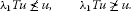In the following, the closed balls in spaces E and $C\left[I,E\right]$ are denoted, respectively, by ${\mathrm{\Omega }}_{l}=\left\{x\in E:\parallel x\parallel \le l\right\}$ ($l>0$) and ${B}_{l}=\left\{x\in C\left[I,E\right]:{\parallel x\parallel }_{C}\le l\right\}$ ($l>0$).

For convenience, let us list the following assumptions:

(${H}_{1}$) $f\in C\left[I×P×P,P\right]$, f is bounded and uniformly continuous in t on $I×{\left(P\cap {\mathrm{\Omega }}_{r}\right)}^{2}$ for any $r>0$, and there exist two nonnegative constants ${l}_{1}$, ${l}_{2}$ with $2{l}_{1}+2{l}_{2}<1$ such that

$\alpha \left(f\left(t×{D}_{1}×{D}_{2}\right)\right)\le {l}_{1}\alpha \left({D}_{1}\right)+{l}_{2}\alpha \left({D}_{2}\right),\phantom{\rule{1em}{0ex}}t\in I,{D}_{1},{D}_{2}\subset P\cap {\mathrm{\Omega }}_{r}.$

(${H}_{2}$) There are three positive constants ${a}_{1}$, ${b}_{1}$, ${c}_{1}$ such that

$\parallel f\left(t,x,y\right)\parallel \le \frac{{a}_{1}\parallel x\parallel +{b}_{1}\parallel y\parallel +{c}_{1}}{N}$

for all $\left(t,x,y\right)\in I×{P}^{2}$ and $\frac{{a}_{1}}{{\pi }^{4}}+\frac{{b}_{1}}{{\pi }^{2}}<1$.

(${H}_{3}$) There is a $\phi \in {P}^{\ast }$ (${P}^{\ast }$ denotes the dual cone of P) with $\phi \left(x\right)>0$ for any $x>\theta$, two nonnegative constants ${a}_{2}$, ${b}_{2}$ and a real number ${r}_{1}>0$ such that

$\phi \left(f\left(t,x,y\right)\right)\ge {a}_{2}\phi \left(x\right)+{b}_{2}\phi \left(y\right)$

for all $\left(t,x,y\right)\in I×{\left(P\cap {\mathrm{\Omega }}_{{r}_{1}}\right)}^{2}$ and $\frac{{a}_{2}}{{\pi }^{4}}+\frac{{b}_{2}}{{\pi }^{2}}>1$.

(${H}_{4}$) There is a $\phi \in {P}^{\ast }$ with $\phi \left(x\right)>0$ for any $x>\theta$ and two nonnegative constants ${a}_{3}$, ${b}_{3}$ and a real number ${R}_{1}>0$ such that

$\phi \left(f\left(t,x,y\right)\right)\ge {a}_{3}\phi \left(x\right)+{b}_{3}\phi \left(y\right),\phantom{\rule{1em}{0ex}}t\in I,x,y\in P,\parallel x\parallel ,\parallel y\parallel \ge {R}_{1}$

and $\frac{{a}_{3}}{{\pi }^{4}}+\frac{{b}_{3}}{{\pi }^{2}}>1$.

(${H}_{5}$) There are three positive constants ${a}_{4}$, ${b}_{4}$, ${r}_{2}$ such that

$\parallel f\left(t,x,y\right)\parallel \le \frac{{a}_{4}\parallel x\parallel +{b}_{4}\parallel y\parallel }{N}$

for all $\left(t,x,y\right)\in I×{\left(P\cap {\mathrm{\Omega }}_{{r}_{2}}\right)}^{2}$ and $\frac{{a}_{4}}{{\pi }^{4}}+\frac{{b}_{4}}{{\pi }^{2}}<1$.

(${H}_{6}$) There exists $\eta >0$ such that

$\underset{t\in I,x,y\in P\cap {\mathrm{\Omega }}_{\eta }}{sup}\parallel f\left(t,x,y\right)\parallel <\frac{4\eta }{N}.$

(${H}_{7}$) There is a $\phi \in {P}^{\ast }$ with $\parallel \phi \parallel =1$ and $\phi \left(x\right)>0$ for any $x>\theta$ and a real number $\eta >0$ such that

$\phi \left(f\left(t,x,y\right)\right)\ge \alpha \eta ,\phantom{\rule{1em}{0ex}}t\in \left[\tau ,1-\tau \right],x,y\in P,\frac{\tau \left(1-\tau \right)\eta }{30N}\le \parallel x\parallel ,\parallel y\parallel \le \eta ,$

where $\tau \in \left(0,\frac{1}{2}\right)$, $\alpha ={\left(\tau \left(1-\tau \right){\int }_{\tau }^{1-\tau }s\left(1-s\right)\phantom{\rule{0.2em}{0ex}}ds\right)}^{-1}$.

Now, let $G\left(t,s\right)$ be the Green’s function of the linear problem ${v}^{″}=0,t\in \left(0,1\right)$ together with $v\left(0\right)=v\left(1\right)=0$, which can be explicitly given by

$G\left(t,s\right)=\left\{\begin{array}{cc}t\left(1-s\right),\hfill & 0\le t\le s\le 1,\hfill \\ s\left(1-t\right),\hfill & 0\le s\le t\le 1.\hfill \end{array}$

Obviously, $G\left(t,s\right)$ have the following properties:

$\begin{array}{r}t\left(1-t\right)s\left(1-s\right)\le G\left(t,s\right)\le t\left(1-t\right),\phantom{\rule{1em}{0ex}}\mathrm{\forall }t,s\in \left[0,1\right];\\ G\left(t,s\right)\ge t\left(1-t\right)G\left(\tau ,s\right),\phantom{\rule{1em}{0ex}}\mathrm{\forall }\tau ,t,s\in \left[0,1\right].\end{array}$
(2.1)

Set

$\left(Su\right)\left(t\right)={\int }_{0}^{1}G\left(t,s\right)u\left(s\right)\phantom{\rule{0.2em}{0ex}}ds,\phantom{\rule{1em}{0ex}}t\in I,u\in C\left[I,E\right].$

Obviously, $S:C\left[I,E\right]\to C\left[I,E\right]$ is continuous.

Let $u=-{x}^{″}$. Since $x\left(0\right)=x\left(1\right)=\theta$, we have

$x\left(t\right)=\left(Su\right)\left(t\right)={\int }_{0}^{1}G\left(t,s\right)u\left(s\right)\phantom{\rule{0.2em}{0ex}}ds.$
(2.2)

Using the above transformation and (2.2), BVP (1.1) becomes

$-{u}^{″}\left(t\right)=f\left(t,\left(Su\right)\left(t\right),u\left(t\right)\right)$
(2.3)

with

$u\left(0\right)=u\left(1\right)=\theta .$
(2.4)

From (2.3) and (2.4), we have

$u\left(t\right)={\int }_{0}^{1}G\left(t,s\right)f\left(s,\left(Su\right)\left(s\right),u\left(s\right)\right)\phantom{\rule{0.2em}{0ex}}ds.$

Now, define an operator A on Q by

$\left(Au\right)\left(t\right)={\int }_{0}^{1}G\left(t,s\right)f\left(s,{\int }_{0}^{1}G\left(s,\tau \right)u\left(\tau \right)\phantom{\rule{0.2em}{0ex}}d\tau ,u\left(s\right)\right)\phantom{\rule{0.2em}{0ex}}ds.$
(2.5)

The following Lemma 2.3 can be easily obtained.

Lemma 2.3 Assume that (${H}_{1}$) holds. Then $A:Q\to {C}^{2}\left[I,P\right]$ and

1. (i)

$A:Q\to Q$ is continuous and bounded;

2. (ii)

BVP (1.1) has a solution in ${C}^{2}\left[I,P\right]\cap {C}^{4}\left[\left(0,1\right),P\right]$ if and only if A has a fixed point in Q.

Let

$\left(T\psi \right)\left(t\right)={\int }_{0}^{1}G\left(t,s\right)\psi \left(s\right)\phantom{\rule{0.2em}{0ex}}ds,\phantom{\rule{1em}{0ex}}\mathrm{\forall }\psi \in C\left[I,\mathbb{R}\right].$

It is easy to see that $T:C\left[I,\mathbb{R}\right]\to C\left[I,\mathbb{R}\right]$ is a ${u}_{0}$-positive operator with ${u}_{0}\left(t\right)=sin\pi t$ and $r\left(T\right)=\frac{1}{{\pi }^{2}}$.

Lemma 2.4 Suppose that (${H}_{1}$) holds. Then for any $l>0$, $A:Q\cap {B}_{l}\to Q$ is a strict set contraction.

Proof For any $u\in Q\cap {B}_{l}$ and $t\in \left[0,1\right]$, by the expression of S, we have

$\parallel \left(Su\right)\left(t\right)\parallel \le {\int }_{0}^{1}G\left(t,s\right)\parallel u\left(s\right)\parallel \phantom{\rule{0.2em}{0ex}}ds\le l,$

and thus $S\left(Q\cap {B}_{l}\right)\subset Q\cap {B}_{l}$ is continuous and bounded. By the uniformly continuous f and (${H}_{1}$), and Lemma 2.1, we have

$\alpha \left(f\left(I×S\left(D\right)×D\right)\right)=\underset{t\in I}{max}\alpha \left(f\left(t×S\left(D\right)×D\right)\right)\le {l}_{1}\alpha \left(S\left(D\right)\right)+{l}_{2}\alpha \left(D\right).$

Since f is uniformly continuous and bounded on $I×{\left(Q\cap {\mathrm{\Omega }}_{l}\right)}^{2}$, we see from (2.5) that A is continuous and bounded on $Q\cap {B}_{l}$. Let $D\subset Q\cap {B}_{l}$, according to (2.5), it is easy to show that the functions $\left\{Au:u\in D\right\}$ are uniformly bounded and equicontinuous, and so in  we have

$\alpha \left(A\left(D\right)\right)=\underset{t\in I}{max}\alpha \left(A\left(D\left(t\right)\right)\right),$

where

Using the obvious formula

${\int }_{0}^{1}u\left(t\right)\phantom{\rule{0.2em}{0ex}}dt\in \overline{\mathit{co}}\left\{u\left(t\right):t\in I\right\},\phantom{\rule{1em}{0ex}}\mathrm{\forall }u\in C\left[I,E\right]$

and observing $0\le G\left(t,s\right)\le 1$, we find

$\begin{array}{rcl}\alpha \left(A\left(D\left(t\right)\right)\right)& \le & \alpha \left(\overline{\mathit{co}}\left\{G\left(t,s\right)f\left(s,Su\left(s\right),u\left(s\right)\right):u\in D,s\in I\right\}\right)\\ \le & \alpha \left(\overline{\mathit{co}}\left\{f\left(s,Su\left(s\right),u\left(s\right)\right)\cup \theta :u\in D,s\in I\right\}\right)\\ =& \alpha \left(\left\{f\left(s,Su\left(s\right),u\left(s\right)\right)\cup \theta :u\in D,s\in I\right\}\right)\\ \le & \alpha \left(\left\{f\left(s,Su\left(s\right),u\left(s\right)\right):u\in D,s\in I\right\}\right)\\ \le & \alpha \left(f\left(I×S\left(B\right)×B\right)\right)\le {l}_{1}\alpha \left(S\left(B\right)\right)+{l}_{2}\alpha \left(B\right)\\ \le & {l}_{1}\alpha \left(B\right)+{l}_{2}\alpha \left(B\right),\end{array}$
(2.6)

where $B=\left\{u\left(s\right)|s\in I,u\in D\right\}\subset {P}_{l}$, $S\left(B\right)=\left\{{\int }_{0}^{1}G\left(t,s\right)u\left(s\right)\phantom{\rule{0.2em}{0ex}}ds|t\in I,u\in D\right\}$.

From the fact of , we know

$\alpha \left(B\right)\le 2\alpha \left(D\right).$
(2.7)

It follows from (2.6) and (2.7) that

$\alpha \left(A\left(D\left(t\right)\right)\right)\le 2\left({l}_{1}+{l}_{2}\right)\alpha \left(D\right),$

and consequently, A is a strict set contraction on $Q\cap {B}_{l}$ because $2\left({l}_{1}+{l}_{2}\right)<1$. □

## 3 Main results

Theorem 3.1 Let a cone P be normal and condition (${H}_{1}$) be satisfied. If (${H}_{2}$) and (${H}_{3}$) or (${H}_{4}$) and (${H}_{5}$) are satisfied, then BVP (1.1) has at least one positive solution.

Proof Set

${Q}_{1}=\left\{u\in Q:u\left(t\right)\ge t\left(1-t\right)u\left(s\right),\mathrm{\forall }t,s\in \left[0,1\right]\right\}.$

It is clear that ${Q}_{1}$ is a cone of the Banach space $C\left[I,E\right]$ and ${Q}_{1}\subset Q$. For any $u\in {Q}_{1}$, by (2.1), we can obtain $A\left(Q\right)\subset {Q}_{1}$, then

$A\left({Q}_{1}\right)\subset {Q}_{1}.$

We first assume that (${H}_{2}$) and (${H}_{3}$) are satisfied. Let

$W=\left\{u\in {Q}_{1}|Au\ge u\right\}.$

In the following, we prove that W is bounded.

For any $u\in W$, we have $\theta \le u\le Au$, that is, $\theta \le u\left(t\right)\le Au\left(t\right)$, $t\in I$. And so $\parallel u\left(t\right)\parallel \le N\parallel Au\left(t\right)\parallel$, set $v\left(t\right)=\parallel u\left(t\right)\parallel$, by (${H}_{2}$)

$\begin{array}{rcl}v\left(t\right)& \le & N\parallel Au\left(t\right)\parallel \\ \le & N{\int }_{0}^{1}G\left(t,s\right)\parallel f\left(s,{\int }_{0}^{1}G\left(s,\tau \right)u\left(\tau \right)\phantom{\rule{0.2em}{0ex}}d\tau ,u\left(s\right)\right)\parallel \phantom{\rule{0.2em}{0ex}}ds\\ \le & {\int }_{0}^{1}G\left(t,s\right)\left({a}_{1}\parallel {\int }_{0}^{1}G\left(s,\tau \right)u\left(\tau \right)\phantom{\rule{0.2em}{0ex}}d\tau \parallel +{b}_{1}\parallel u\left(s\right)\parallel +{c}_{1}\right)\phantom{\rule{0.2em}{0ex}}ds\\ \le & {\int }_{0}^{1}G\left(t,s\right)\left({a}_{1}{\int }_{0}^{1}G\left(s,\tau \right)\parallel u\left(\tau \right)\parallel \phantom{\rule{0.2em}{0ex}}d\tau +{b}_{1}\parallel u\left(s\right)\parallel \right)\phantom{\rule{0.2em}{0ex}}ds+{c}_{1}.\end{array}$
(3.1)

For $\psi \in C\left[I,\mathbb{R}\right]$, let ${L}_{1}\psi ={a}_{1}{T}^{2}\psi +{b}_{1}T\psi$, then ${L}_{1}:C\left[I,\mathbb{R}\right]\to C\left[I,\mathbb{R}\right]$ is a bounded linear operator. From (3.1), one deduces that

$\left(\left(I-{L}_{1}\right)v\right)\left(t\right)\le {c}_{1}.$

Since ${\pi }^{2}$ is the first eigenvalue of T, by (${H}_{2}$), the first eigenvalue of ${L}_{1}$, $r\left({L}_{1}\right)=\frac{{a}_{1}}{{\pi }^{4}}+\frac{{b}_{1}}{{\pi }^{2}}<1$. Therefore, by , the inverse operator ${\left(I-{L}_{1}\right)}^{-1}$ exists and

${\left(I-{L}_{1}\right)}^{-1}=I+{L}_{1}+{L}_{1}^{2}+\cdots +{L}_{1}^{n}+\cdots .$

It follows from ${L}_{1}\left(K\right)\subset K$ that ${\left(I-{L}_{1}\right)}^{-1}\left(K\right)\subset K$. So, we know that $v\left(t\right)\le {\left(I-{L}_{1}\right)}^{-1}{c}_{1}$, $t\in \left[0,1\right]$ and W is bounded.

Taking ${R}_{2}>max\left\{{r}_{1},supW\right\}$, we have

$Au\ngeqq u,\phantom{\rule{1em}{0ex}}\mathrm{\forall }u\in \partial {B}_{{R}_{2}}\cap {Q}_{1}.$
(3.2)

Next, we are going to verify that for any ${r}_{3}\in \left(0,{r}_{1}\right)$,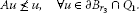(3.3)

If this is false, then there exists ${u}_{1}\in \partial {B}_{{r}_{3}}\cap {Q}_{1}$ such that $A{u}_{1}\le {u}_{1}$. This together with (${H}_{3}$) yields

$\begin{array}{rcl}\phi \left({u}_{1}\left(t\right)\right)& \ge & \phi \left(\left(A{u}_{1}\right)\left(t\right)\right)=\phi \left({\int }_{0}^{1}G\left(t,s\right)f\left(s,\left(S{u}_{1}\right)\left(s\right),{u}_{1}\left(s\right)\right)\phantom{\rule{0.2em}{0ex}}ds\right)\\ =& {\int }_{0}^{1}G\left(t,s\right)\phi \left(f\left(s,\left(S{u}_{1}\right)\left(s\right),{u}_{1}\left(s\right)\right)\right)\phantom{\rule{0.2em}{0ex}}ds\\ \ge & {\int }_{0}^{1}G\left(t,s\right)\left({a}_{2}\phi \left(\left(S{u}_{1}\right)\left(s\right)\right)+{b}_{2}\phi \left({u}_{1}\left(s\right)\right)\right)\phantom{\rule{0.2em}{0ex}}ds\\ =& {\int }_{0}^{1}G\left(t,s\right)\left({a}_{2}{\int }_{0}^{1}G\left(s,\tau \right)\phi \left({u}_{1}\left(\tau \right)\right)\phantom{\rule{0.2em}{0ex}}d\tau +{b}_{2}\phi \left({u}_{1}\left(s\right)\right)\right)\phantom{\rule{0.2em}{0ex}}ds\\ =& \left({a}_{2}{T}^{2}+{b}_{2}T\right)\phi \left({u}_{1}\left(t\right)\right),\phantom{\rule{1em}{0ex}}t\in I.\end{array}$

For $\psi \in C\left[I,\mathbb{R}\right]$, let ${L}_{2}\psi ={a}_{2}{T}^{2}\psi +{b}_{2}T\psi$, then the above inequality can be written in the form

$\phi \left({u}_{1}\left(t\right)\right)\ge {L}_{2}\left(\phi \left({u}_{1}\left(t\right)\right)\right).$
(3.4)

It is easy to see that

$\phi \left({u}_{1}\left(t\right)\right)\ne 0,\phantom{\rule{1em}{0ex}}t\in I.$

In fact, $\phi \left({u}_{1}\left(t\right)\right)=0$ implies ${u}_{1}\left(t\right)=\theta$ for $t\in I$, and consequently, ${\parallel {u}_{1}\parallel }_{C}=0$ in contradiction to ${\parallel {u}_{1}\parallel }_{C}={r}_{3}$. Now, notice that ${L}_{2}$ is a ${u}_{0}$-positive operator with ${u}_{0}\left(t\right)=sin\pi t$, then by Lemma 2.2, we have $\phi \left({u}_{1}\left(t\right)\right)={\mu }_{0}sin\pi t$ for some ${\mu }_{0}>0$. This together with $\left(\frac{{a}_{2}}{{\pi }^{4}}+\frac{{b}_{2}}{{\pi }^{2}}\right)sin\pi t={L}_{2}\left(sin\pi t\right)$ and (3.4) implies that

${\mu }_{0}sin\pi t=\phi \left({u}_{1}\left(t\right)\right)\ge {L}_{2}\left(\phi \left({u}_{1}\left(t\right)\right)\right)={L}_{2}\left({\mu }_{0}sin\pi t\right)={\mu }_{0}\left(\frac{{a}_{2}}{{\pi }^{4}}+\frac{{b}_{2}}{{\pi }^{2}}\right)sin\pi t,$

which is a contradiction to $\frac{{a}_{2}}{{\pi }^{4}}+\frac{{b}_{2}}{{\pi }^{2}}>1$. So, (3.3) holds.

By Lemma 2.4, A is a strict set contraction on ${Q}_{{r}_{3},{R}_{2}}=\left\{u\in {Q}_{1}:{r}_{3}\le {\parallel u\parallel }_{C}\le {R}_{2}\right\}$. Observing (3.2) and (3.3) and using Theorem 2.1, we see that A has a fixed point on ${Q}_{{r}_{3},{R}_{2}}$.

Next, in the case that (${H}_{4}$) and (${H}_{5}$) are satisfied, by the method as in establishing (3.3), we can assert from (${H}_{5}$) that for any ${r}_{4}\in \left(0,{r}_{2}\right)$,

$Au\ngeqq u,\phantom{\rule{1em}{0ex}}\mathrm{\forall }u\in \partial {B}_{{r}_{4}}\cap {Q}_{1}.$
(3.5)

Let

$\left({L}_{3}v\right)\left(t\right)={\int }_{0}^{1}G\left(t,s\right)\left({a}_{3}{\int }_{0}^{1}G\left(s,\tau \right)v\left(\tau \right)\phantom{\rule{0.2em}{0ex}}d\tau +{b}_{3}v\left(s\right)\right)\phantom{\rule{0.2em}{0ex}}ds,\phantom{\rule{1em}{0ex}}v\in C\left[I,\mathbb{R}\right].$

It is clear that ${L}_{3}:C\left[I,\mathbb{R}\right]\to C\left[I,\mathbb{R}\right]$ is a completely continuous linear ${u}_{0}$-operator with ${u}_{0}\left(t\right)=sin\pi t$ and ${L}_{3}:{K}_{1}\to {K}_{1}$ in which ${K}_{1}=\left\{v\in K\subset C\left[I,\mathbb{R}\right]:v\left(t\right)\ge t\left(1-t\right)v\left(s\right),\mathrm{\forall }t,s\in I\right\}$. In addition, the spectral radius $r\left({L}_{3}\right)=\frac{{a}_{3}}{{\pi }^{4}}+\frac{{b}_{3}}{{\pi }^{2}}$ and $sin\pi t$ is the positive eigenfunction of ${L}_{3}$ corresponding to its first eigenvalue ${\lambda }_{1}={\left(r\left({L}_{3}\right)\right)}^{-1}$.

Let

$\left({L}_{\delta }v\right)\left(t\right)={\int }_{\delta }^{1-\delta }G\left(t,s\right)\left({a}_{3}{\int }_{0}^{1}G\left(s,\tau \right)v\left(\tau \right)\phantom{\rule{0.2em}{0ex}}d\tau +{b}_{3}v\left(s\right)\right)\phantom{\rule{0.2em}{0ex}}ds,\phantom{\rule{1em}{0ex}}v\in C\left[I,\mathbb{R}\right],$

where $\delta \in \left(0,\frac{1}{2}\right)$. It is clear that ${L}_{\delta }:C\left[I,\mathbb{R}\right]\to C\left[I,\mathbb{R}\right]$ is a completely continuous linear ${u}_{0}$-operator with ${u}_{0}\left(t\right)=t\left(1-t\right)$ and ${L}_{\delta }\left({K}_{1}\right)\subset {K}_{1}$. Thus, the spectral radius $r\left({L}_{\delta }\right)\ne 0$ and ${L}_{\delta }$ has a positive eigenfunction corresponding to its first eigenvalue ${\lambda }_{\delta }={\left(r\left({L}_{\delta }\right)\right)}^{-1}$.

Take ${\delta }_{n}\in \left(0,1/2\right)$ ($n=1,2,\dots$) satisfying ${\delta }_{1}\ge {\delta }_{2}\ge \cdots \ge {\delta }_{n}\ge \cdots$ and ${\delta }_{n}\to 0$ ($n\to \mathrm{\infty }$). For $m>n$, $v\in {K}_{1}$, we have

$\left({L}_{{\delta }_{n}}v\right)\left(t\right)\le \left({L}_{{\delta }_{m}}v\right)\left(t\right)\le \left({L}_{3}v\right)\left(t\right),\phantom{\rule{1em}{0ex}}\mathrm{\forall }t\in I.$

By , we have $r\left({L}_{{\delta }_{n}}\right)\le r\left({L}_{{\delta }_{m}}\right)\le r\left({L}_{3}\right)$. Let ${\lambda }_{{\delta }_{n}}={\left(r\left({T}_{{\delta }_{n}}\right)\right)}^{-1}$, by Gelfand’s formula, we have ${\lambda }_{{\delta }_{n}}\ge {\lambda }_{{\delta }_{m}}\ge {\lambda }_{1}$. Let ${\lambda }_{{\delta }_{n}}\to {\stackrel{˜}{\lambda }}_{1}$ as $n\to \mathrm{\infty }$.

In the following, we prove that ${\stackrel{˜}{\lambda }}_{1}={\lambda }_{1}$.

Let ${v}_{{\delta }_{n}}$ be the positive eigenfunction of ${T}_{{\delta }_{n}}$ corresponding to ${\lambda }_{{\delta }_{n}}$, i.e.,

$\begin{array}{rcl}{v}_{{\delta }_{n}}\left(t\right)& =& {\lambda }_{{\delta }_{n}}\left({L}_{\delta }{v}_{{\delta }_{n}}\right)\left(t\right)\\ =& {\lambda }_{{\delta }_{n}}{\int }_{{\delta }_{n}}^{1-{\delta }_{n}}G\left(t,s\right)\left({a}_{3}{\int }_{0}^{1}G\left(s,\tau \right){v}_{{\delta }_{n}}\left(\tau \right)\phantom{\rule{0.2em}{0ex}}d\tau +{b}_{3}{v}_{{\delta }_{n}}\left(s\right)\right)\phantom{\rule{0.2em}{0ex}}ds,\end{array}$
(3.6)

satisfying $\parallel {v}_{{\delta }_{n}}\parallel =1$. Without loss of generality, by standard argument, we may suppose by the Arzela-Ascoli theorem and ${\lambda }_{{\delta }_{n}}\to {\stackrel{˜}{\lambda }}_{1}$ that ${v}_{{\delta }_{n}}\to {\stackrel{˜}{v}}_{0}$ as $n\to \mathrm{\infty }$. Thus, $\parallel {\stackrel{˜}{v}}_{0}\parallel =1$ and by (3.6), we have

${\stackrel{˜}{v}}_{0}\left(t\right)={\stackrel{˜}{\lambda }}_{1}{\int }_{0}^{1}G\left(t,s\right)\left({a}_{3}{\int }_{0}^{1}G\left(s,\tau \right){\stackrel{˜}{v}}_{0}\left(\tau \right)\phantom{\rule{0.2em}{0ex}}d\tau +{b}_{3}{\stackrel{˜}{v}}_{0}\left(s\right)\right)\phantom{\rule{0.2em}{0ex}}ds,$

that is, ${\stackrel{˜}{v}}_{0}\left(t\right)={\stackrel{˜}{\lambda }}_{1}\left({L}_{3}{\stackrel{˜}{v}}_{0}\right)\left(t\right)$. This together with Lemma 2.2 guarantees that ${\stackrel{˜}{\lambda }}_{1}={\lambda }_{1}$.

By the above argument, it is easy to see that there exists a $\delta \in \left(0,\frac{1}{2}\right)$ such that

$1

Choose

${R}_{3}>max\left\{{R}_{1},{r}_{2},\frac{30N{R}_{1}}{\delta \left(1-\delta \right)}\right\}.$
(3.7)

Now, we assert that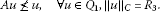(3.8)

If this is not true, then there exists ${u}_{2}\in {Q}_{1}$ with ${\parallel u\parallel }_{C}={R}_{3}$ such that $A{u}_{2}\le {u}_{2}$, then $A{u}_{2}\left(t\right)\le {u}_{2}\left(t\right)$. Moreover, by the definition of ${Q}_{1}$, we know

$\begin{array}{c}{u}_{2}\left(t\right)\ge t\left(1-t\right){u}_{2}\left(s\right),\phantom{\rule{1em}{0ex}}\mathrm{\forall }t,s\in I,\hfill \\ \left(S{u}_{2}\right)\left(t\right)={\int }_{0}^{1}G\left(t,s\right){u}_{2}\left(s\right)\phantom{\rule{0.2em}{0ex}}ds\ge t\left(1-t\right){\int }_{0}^{1}{s}^{2}{\left(1-s\right)}^{2}\phantom{\rule{0.2em}{0ex}}ds\cdot {u}_{2}\left(\tau \right),\phantom{\rule{1em}{0ex}}\mathrm{\forall }t,\tau \in I.\hfill \end{array}$

Thus, $N\parallel {u}_{2}\left(t\right)\parallel \ge t\left(1-t\right){\parallel {u}_{2}\parallel }_{C}$, $N\parallel \left(S{u}_{2}\right)\left(t\right)\parallel \ge \frac{t\left(1-t\right)}{30}{\parallel {u}_{2}\parallel }_{C}$, which implies by (3.7) we have

$\underset{t\in \left[\delta ,1-\delta \right]}{min}\parallel u\left(t\right)\parallel \ge \frac{\delta \left(1-\delta \right)}{N}{\parallel u\parallel }_{C}\ge {R}_{1},$

and

$\underset{t\in \left[\delta ,1-\delta \right]}{min}\parallel \left(Su\right)\left(t\right)\parallel \ge \frac{\delta \left(1-\delta \right)}{30N}{\parallel u\parallel }_{C}\ge {R}_{1}.$

So, by (${H}_{4}$), we get

$\begin{array}{rcl}\phi \left({u}_{2}\left(t\right)\right)& \ge & \phi \left(\left(A{u}_{2}\right)\left(t\right)\right)\\ =& \phi \left({\int }_{0}^{1}G\left(t,s\right)f\left(s,\left(S{u}_{2}\right)\left(s\right),{u}_{2}\left(s\right)\right)\phantom{\rule{0.2em}{0ex}}ds\right)\\ \ge & {\int }_{\delta }^{1-\delta }G\left(t,s\right)\phi \left(f\left(s,\left(S{u}_{2}\right)\left(s\right),{u}_{2}\left(s\right)\right)\right)\phantom{\rule{0.2em}{0ex}}ds\\ \ge & {\int }_{\delta }^{1-\delta }G\left(t,s\right)\left({a}_{3}\phi \left(\left(S{u}_{2}\right)\left(s\right)\right)+{b}_{3}\phi \left({u}_{2}\left(s\right)\right)\right)\phantom{\rule{0.2em}{0ex}}ds\\ \ge & {\int }_{\delta }^{1-\delta }G\left(t,s\right)\left({a}_{3}{\int }_{0}^{1}G\left(s,\tau \right)\phi \left({u}_{2}\left(\tau \right)\right)\phantom{\rule{0.2em}{0ex}}d\tau +{b}_{3}\phi \left({u}_{2}\left(s\right)\right)\right)\phantom{\rule{0.2em}{0ex}}ds\\ =& {L}_{\delta }\left(\phi \left({u}_{2}\left(t\right)\right)\right).\end{array}$

It is easy to see that

$\phi \left({u}_{2}\left(t\right)\right)\ne 0,\phantom{\rule{1em}{0ex}}t\in I.$

In fact, $\phi \left({u}_{2}\left(t\right)\right)=0$ implies ${u}_{2}\left(t\right)=\theta$ for $t\in I$, and consequently, ${\parallel {u}_{2}\parallel }_{C}=0$ in contradiction to ${\parallel {u}_{2}\parallel }_{C}={R}_{3}$. Now, notice that ${L}_{\delta }$ is a ${u}_{0}$-positive operator with ${u}_{0}\left(t\right)=t\left(1-t\right)$. Then by Lemma 2.2, we have $\phi \left({u}_{2}\left(t\right)\right)={\mu }_{0}{v}_{\delta }\left(t\right)$ for some ${\mu }_{0}>0$, where ${v}_{\delta }$ is the positive eigenfunction of ${L}_{\delta }$ corresponding to ${\lambda }_{\delta }$. This together with $r\left({L}_{\delta }\right){v}_{\delta }\left(t\right)={L}_{\delta }\left({v}_{\delta }\left(t\right)\right)$ implies that

${\mu }_{0}{v}_{\delta }\left(t\right)=\phi \left({u}_{2}\left(t\right)\right)\ge {L}_{\delta }\left(\phi \left({u}_{2}\left(t\right)\right)\right)={L}_{3}\left({\mu }_{0}{v}_{\delta }\left(t\right)\right)={\mu }_{0}r\left({L}_{\delta }\right){v}_{\delta }\left(t\right),$

which is a contradiction to $r\left({L}_{\delta }\right)>1$. So, (3.8) holds.

By Lemma 2.4, A is a strict set contraction on ${Q}_{{r}_{4},{R}_{3}}=\left\{u\in {Q}_{1}:{r}_{4}\le {\parallel u\parallel }_{C}\le {R}_{3}\right\}$. Observing (3.5) and (3.8) and using Theorem 2.1, we see that A has a fixed point on ${Q}_{{r}_{4},{R}_{3}}$. This together with Lemma 2.3 implies that BVP (1.1) has at least one positive solution. □

Theorem 3.2 Let a cone P be normal. Suppose that conditions (${H}_{1}$), (${H}_{3}$), (${H}_{4}$) and (${H}_{6}$) are satisfied. Then BVP (1.1) has at least two positive solutions.

Proof We can take the same ${Q}_{1}\subset C\left[I,E\right]$ as in Theorem 3.1. As in the proof of Theorem 3.1, we can also obtain that $A\left({Q}_{1}\right)\subset {Q}_{1}$. And we choose ${r}_{5}$, ${R}_{4}$ with ${R}_{4}>\eta >{r}_{5}>0$ such that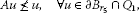(3.9)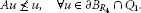(3.10)

On the other hand, it is easy to see that

$Au\ngeqq u,\phantom{\rule{1em}{0ex}}\mathrm{\forall }u\in \partial {B}_{\eta }\cap {Q}_{1}.$
(3.11)

In fact, if there exists ${u}_{3}\in {Q}_{1}$ with ${\parallel {u}_{3}\parallel }_{C}=\eta$ such that $A{u}_{3}\ge {u}_{3}$, then observing ${max}_{t,s\in I}G\left(t,s\right)=\frac{1}{4}$ and ${\parallel S{u}_{2}\parallel }_{C}\le \eta$, we get

$\begin{array}{rcl}\theta & \le & {u}_{3}\left(t\right)\le {\int }_{0}^{1}G\left(t,s\right)f\left(s,\left(S{u}_{3}\right)\left(s\right),{u}_{3}\left(s\right)\right)\phantom{\rule{0.2em}{0ex}}ds\\ \le & \frac{1}{4}{\int }_{0}^{1}f\left(s,\left(S{u}_{3}\right)\left(s\right),{u}_{3}\left(s\right)\right)\phantom{\rule{0.2em}{0ex}}ds,\phantom{\rule{1em}{0ex}}\mathrm{\forall }t\in I,\end{array}$

and so

$\parallel {u}_{3}\left(t\right)\parallel \le \frac{N}{4}{\int }_{0}^{1}\parallel f\left(s,\left(S{u}_{3}\right)\left(s\right),{u}_{3}\left(s\right)\right)\parallel \phantom{\rule{0.2em}{0ex}}ds\le \frac{1}{4}NM,\phantom{\rule{1em}{0ex}}t\in I,$
(3.12)

where, by virtue of (${H}_{5}$),

$M=\underset{t\in I,x,y\in P\cap {\mathrm{\Omega }}_{\eta }}{sup}\parallel f\left(t,x,y\right)\parallel <\frac{4\eta }{N}.$
(3.13)

It follows from (3.12) and (3.13) that

$\eta ={\parallel {u}_{3}\parallel }_{C}\le \frac{4\eta }{N}M<\eta ,$

a contradiction. Thus (3.11) is true.

By Lemma 2.4, A is a strict set contraction on ${Q}_{{r}_{5},\eta }=\left\{u\in {Q}_{1}|{r}_{5}\le {\parallel u\parallel }_{C}\le \eta \right\}$, and also on ${Q}_{\eta ,{R}_{4}}=\left\{u\in {Q}_{1}|\eta \le {\parallel u\parallel }_{C}\le {R}_{4}\right\}$. Observing (3.9), (3.10), (3.11) and applying, respectively, Theorem 2.1 to A, ${Q}_{{r}_{5},\eta }$ and ${Q}_{\eta ,{R}_{4}}$, we assert that there exist ${u}_{1}\in {Q}_{{r}_{5},\eta }$ and ${u}_{2}\in {Q}_{\eta ,{R}_{4}}$ such that $A{u}_{1}={u}_{1}$ and $A{u}_{2}={u}_{2}$ and, by Lemma 2.3 and (3.11), $S{u}_{1}$, $S{u}_{2}$ are positive solutions of BVP (1.1). □

Theorem 3.3 Let a cone P be normal. Suppose that conditions (${H}_{1}$), (${H}_{2}$) and (${H}_{5}$) and (${H}_{7}$) are satisfied. Then BVP (1.1) has at least two positive solutions.

Proof We can take the same ${Q}_{1}\subset C\left[I,E\right]$ as in Theorem 3.1. As in the proof of Theorem 3.1, we can also obtain that $A\left({Q}_{1}\right)\subset {Q}_{1}$. And we choose ${r}_{6}$, ${R}_{5}$ with ${R}_{5}>\eta >{r}_{6}>0$ such that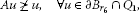(3.14)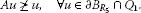(3.15)

On the other hand, it is easy to see that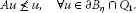(3.16)

In fact, if there exists ${u}_{4}\in {Q}_{1}$ with ${\parallel {u}_{4}\parallel }_{C}=\eta$ such that $A{u}_{4}\ge {u}_{4}$, then

$\theta \le \left(A{u}_{4}\right)\left(t\right)\le {u}_{4}\left(t\right),\phantom{\rule{1em}{0ex}}t\in I.$

Observing ${u}_{4}\left(t\right)\in {Q}_{1}$ and $\frac{t\left(1-t\right)}{30}{u}_{4}\left(s\right)\le \left(S{u}_{4}\right)\left(t\right)\le {u}_{4}\left(s\right)$, we get

$\begin{array}{rcl}\eta & =& \parallel \phi \parallel {\parallel {u}_{4}\parallel }_{C}\ge \phi \left({u}_{4}\left(t\right)\right)\ge \phi \left(\left(A{u}_{4}\right)\left(t\right)\right)\\ =& {\int }_{0}^{1}G\left(t,s\right)\phi \left(f\left(s,\left(S{u}_{4}\right)\left(s\right),{u}_{4}\left(s\right)\right)\right)\phantom{\rule{0.2em}{0ex}}ds\\ \ge & {\int }_{\tau }^{1-\tau }G\left(t,s\right)\phi \left(f\left(s,\left(S{u}_{4}\right)\left(s\right),{u}_{4}\left(s\right)\right)\right)\phantom{\rule{0.2em}{0ex}}ds\\ >& \alpha \eta \tau \left(1-\tau \right){\int }_{\tau }^{1-\tau }s\left(1-s\right)\phantom{\rule{0.2em}{0ex}}ds=\eta ,\end{array}$

which is a contradiction. Hence, (3.16) holds.

By Lemma 2.4, A is a strict set contraction on ${Q}_{{r}_{6},\eta }=\left\{u\in {Q}_{1}|{r}_{6}\le {\parallel u\parallel }_{C}\le \eta \right\}$ and also on ${Q}_{\eta ,{R}_{5}}=\left\{u\in {Q}_{1}|\eta \le {\parallel u\parallel }_{C}\le {R}_{5}\right\}$. Observing (3.14), (3.15), (3.16) and applying, respectively, Theorem 2.1 to A, ${Q}_{{r}_{6},\eta }$ and ${Q}_{\eta ,{R}_{5}}$, we assert that there exist ${u}_{1}\in {Q}_{{r}_{6},\eta }$ and ${u}_{2}\in {Q}_{\eta ,{R}_{5}}$ such that $A{u}_{1}={u}_{1}$ and $A{u}_{2}={u}_{2}$ and, by Lemma 2.3 and (3.16), $S{u}_{1}$, $S{u}_{2}$ are positive solutions of BVP (1.1). □

## 4 One example

Now, we consider an example to illustrate our results.

Example 4.1 Consider the following boundary value problem of the finite system of scalar differential equations:

$\left\{\begin{array}{c}{x}_{n}^{\left(4\right)}\left(t\right)={f}_{n}\left(t,x\left(t\right),-{x}^{″}\left(t\right)\right),\phantom{\rule{1em}{0ex}}t\in \left(0,1\right),\hfill \\ x\left(0\right)=x\left(1\right)={x}^{″}\left(0\right)={x}^{″}\left(1\right)=0,\hfill \end{array}$
(4.1)

where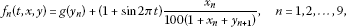(4.2)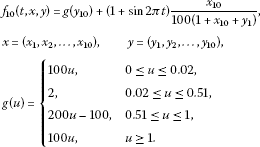(4.3)

Claim (4.1) has at least two positive solutions ${x}^{\ast }\left(t\right)=\left({x}_{1}^{\ast }\left(t\right),{x}_{2}^{\ast }\left(t\right),\dots ,{x}_{10}^{\ast }\left(t\right)\right)$ and ${x}^{\ast \ast }\left(t\right)=\left({x}_{1}^{\ast \ast }\left(t\right),{x}_{2}^{\ast \ast }\left(t\right),\dots ,{x}_{10}^{\ast \ast }\left(t\right)\right)$ such that

$0<\underset{1\le i\le 10,t\in \left[0,1\right]}{max}|{x}_{i}^{\ast }\left(t\right)|<0.51<\underset{1\le i\le 10,t\in \left[0,1\right]}{max}|{x}_{i}^{\ast \ast }\left(t\right)|.$

Proof Let $E={\mathbb{R}}^{10}=\left\{x=\left({x}_{1},{x}_{2},\dots ,{x}_{10}\right):{x}_{n}\in \mathbb{R},n=1,2,\dots ,10\right\}$ with the norm $\parallel x\parallel ={max}_{1\le n\le 10}|{x}_{n}|$, and $P=\left\{x=\left({x}_{1},{x}_{2},\dots ,{x}_{10}\right):{x}_{n}\ge 0,n=1,2,\dots ,10\right\}$. Then P is a normal cone in E, and the normal constant is $N=1$. System (4.1) can be regarded as a boundary value problem of (1.1) in E, where $x=\left({x}_{1},{x}_{2},\dots ,{x}_{10}\right)$, $y=\left({y}_{1},{y}_{2},\dots ,{y}_{10}\right)$,

$f\left(t,x,y\right)=\left({f}_{1}\left(t,x,y\right),{f}_{2}\left(t,x,y\right),\dots ,{f}_{10}\left(t,x,y\right)\right).$

Evidently, $f:I×{P}^{2}\to P$ is continuous. In this case, condition (${H}_{1}$) is automatically satisfied. Since $\alpha \left(f\left(t,{D}_{1},{D}_{2}\right)\right)$ is identical zero for any $t\in I$ and ${D}_{1},{D}_{2}\subset P\cap {\mathrm{\Omega }}_{l}$. Obviously, ${P}^{\ast }=P$, so we may choose $\phi =\left(1,1,\dots ,1\right)$, then for any $x,y\in P$, we have

$\phi \left(f\left(t,x,y\right)\right)=\sum _{n=1}^{10}{f}_{n}\left(t,x,y\right).$

Noticing $\parallel x\parallel \le \phi \left(x\right)\le 10\parallel x\parallel$, we have

$\phi \left(f\left(t,x,y\right)\right)=\sum _{n=1}^{10}{f}_{n}\left(t,x,y\right)\ge 100\parallel y\parallel \ge 10\phi \left(y\right)$

for all $\left(t,x,y\right)\in I×{P}^{2}$ with $\parallel x\parallel \le 0.02$ and $\parallel y\parallel \le 0.02$, and

$\phi \left(f\left(t,x,y\right)\right)=\sum _{n=1}^{10}{f}_{n}\left(t,x,y\right)\ge 100\parallel y\parallel \ge 10\phi \left(y\right),$

for all $\left(t,x,y\right)\in I×{P}^{2}$ with $\parallel x\parallel \ge 1$ and $\parallel y\parallel \ge 1$. So, the conditions (${H}_{3}$) and (${H}_{4}$) are satisfied with ${a}_{2}={a}_{3}=0$ and ${b}_{2}={b}_{3}=10$.

Choosing $\eta =0.51$ for $t\in I$ and $x,y\in P$ with $\parallel x\parallel ,\parallel y\parallel \le 0.51$, we have

$\parallel f\left(t,x,y\right)\parallel \le 2.0102<2.04=4\eta .$

So, condition (${H}_{6}$) is satisfied. Thus, our conclusion follows from Theorem 3.2. □

## References

1. Aftabizadeh AR: Existence and uniqueness theorems for fourth-order boundary value problems. J. Math. Anal. Appl. 1986, 116: 415-426. 10.1016/S0022-247X(86)80006-3

2. Yang Y: Fourth-order two-point boundary value problems. Proc. Am. Math. Soc. 1988, 104: 175-180. 10.1090/S0002-9939-1988-0958062-3

3. del Pino MA, Manasevich RF: Existence for a fourth-order boundary value problem under a two-parameter nonresonance condition. Proc. Am. Math. Soc. 1991, 112: 81-86.

4. Agarwal RP: On fourth-order boundary value problems arising in beam analysis. Differ. Integral Equ. 1989, 2: 91-110.

5. Cui Y, Zou Y: Existence and uniqueness theorems for fourth-order singular boundary value problems. Comput. Math. Appl. 2009, 58: 1449-1456. 10.1016/j.camwa.2009.07.041

6. Deimling K: Ordinary Differential Equations in Banach Spaces. Springer, Berlin; 1977.

7. Lakshmikantham V, Leela S: Nonlinear Differential Equations in Abstract Spaces. Pergamon, Oxford; 1981.

8. Guo D, Lakshmikantham V, Liu X: Nonlinear Integral Equations in Abstract Spaces. Kluwer Academic, Dordrecht; 1996.

9. Guo D, Lakshmikantham V: Multiple solutions of two-point boundary value problem of ordinary differential equations in Banach space. J. Math. Anal. Appl. 1988, 129: 211-222. 10.1016/0022-247X(88)90243-0

10. Liu Y: Multiple positive solutions to fourth-order singular boundary value problems in abstract space. Electron. J. Differ. Equ. 2004, 120: 1-13.

11. Zhang X, Feng M, Ge W: Existence results for nonlinear boundary value problems with integral boundary conditions in Banach spaces. Nonlinear Anal. 2008, 69: 3310-3321. 10.1016/j.na.2007.09.020

12. Krasnosel’skii MA: Positive Solutions of Operator Equations. Noordhoff, Groningen; 1964.

13. Krasnosel’skii MA, Zabreiko PP: Geometrical Methods of Nonlinear Analysis. Springer, Berlin; 1984.

14. Xia D, Wu Z, Yan S, Shu W: Theory of Real Variable Functions and Functional Analysis. 2nd edition. Higher Education Press, Beijing; 1985.

## Acknowledgements

The authors would like to thank the referees for carefully reading this article and making valuable comments and suggestions. This work is supported by the Foundation items: NSFC (10971179), NSF (BS2010SF023, BS2012SF022) of Shandong Province.

## Author information

Authors

### Corresponding author

Correspondence to Yujun Cui.

### Competing interests

The authors declare that they have no competing interests.

### Authors’ contributions

All authors typed, read and approved the final manuscript.

## Rights and permissions

Reprints and Permissions

Cui, Y., Sun, J. Existence of multiple positive solutions for fourth-order boundary value problems in Banach spaces. Bound Value Probl 2012, 107 (2012). https://doi.org/10.1186/1687-2770-2012-107

• Accepted:

• Published:

• DOI: https://doi.org/10.1186/1687-2770-2012-107

### Keywords

• ${u}_{0}$-positive operator
• boundary value problem
• positive solution
• fixed-point theorem
• measure of noncompactness